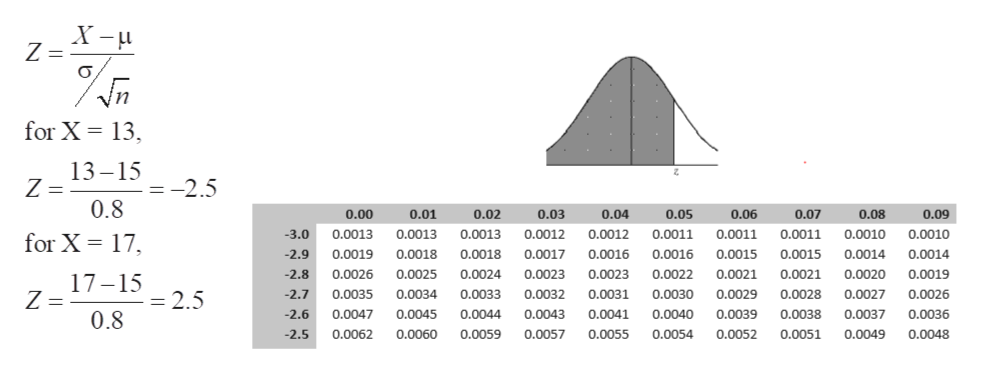# Let x represent the dollar amount spent on supermarket impulse buying in a 10-minute (unplanned) shopping interval. Based on a certain article, the mean of the x distribution is about \$15 and the estimated standard deviation is about \$8.Consider a random sample of n = 100 customers, each of whom has 10 minutes of unplanned shopping time in a supermarket. From the central limit theorem, what can you say about the probability distribution of x, the average amount spent by these customers due to impulse buying? What are the mean and standard deviation of the x distribution?A) What is the probability that x is between \$13 and \$17? (Round your answer to four decimal places.B)  Let us assume that x has a distribution that is approximately normal. What is the probability that x is between \$13 and \$17? (Round your answer to four decimal places.

Question
603 views

Let x represent the dollar amount spent on supermarket impulse buying in a 10-minute (unplanned) shopping interval. Based on a certain article, the mean of the x distribution is about \$15 and the estimated standard deviation is about \$8.

Consider a random sample of n = 100 customers, each of whom has 10 minutes of unplanned shopping time in a supermarket. From the central limit theorem, what can you say about the probability distribution of x, the average amount spent by these customers due to impulse buying? What are the mean and standard deviation of the x distribution?

A) What is the probability that x is between \$13 and \$17? (Round your answer to four decimal places.

B)  Let us assume that x has a distribution that is approximately normal. What is the probability that x is between \$13 and \$17? (Round your answer to four decimal places.

check_circle

Step 1

given x represent the dollar amount spent on supermarket impulse buying in a 10-minute shopping interval.
Mean of x distribution is \$15 and standard deviation of x distribution is \$8.
Sample size = n = 8.

According to central limit theorem states that given a sufficiently large sample size from a population with a finite level of variance, the mean of all samples from the same population will be approximately equal to the mean of the population.

So we can say the average amount spent by the customers due to impulse buying will be normal distribution (as sample size is large 100) with mean same aspopulation mean and standard deviation is   σ/√n, where   σ is population standard deviation and n is sample size. So by substituting values we get sample mean as \$15 and sample standard deviation as 0.8 as shown below

Step 2

The Z score is the number of standard deviaiton a point is from the mean of the distribution, it is calculated by below mentioned formula. By substituting values x = 13, 17 we get their respective Z scores as shown below.

P(13 ≤ x ≤ 17) = P( -2.5 ≤ Z ≤  2.5)

With respect to below shown Z table we can see that P( ...help_outlineImage TranscriptioncloseZ = Vn for X 13 13-15 = Z 0.8 =-2.5 0.00 0.01 0.03 0.05 0.06 0.07 0.08 0.09 0.02 0.04 -3.0 0.0013 0.0013 0.0013 0.0012 0.0012 0.0011 0.0011 0.0011 0.0010 0.0010 for X 17 -2.9 0.0019 0.0018 0.0018 0.0017 0.0016 0.0016 0.0015 0.0015 0.0014 0.0014 -2.8 0.0026 0.0025 0.0024 0.0023 0.0023 0.0022 0.0021 0.0021 0.0020 0.0019 17-15 = 2.5 -2.7 0.0033 0.0031 0.0030 0.0029 0.0028 0.0027 0.0026 Z = 0.0035 0.0034 0.0032 -2.6 0.0047 0.0045 0.0044 0.0043 0.0041 0.0040 0.0039 0.0038 0.0037 0.0036 0.8 -2.5 0.0062 0.0060 0.0059 0.0057 0.0055 0.0054 0.0052 0.0051 0.0049 0.0048 fullscreen

### Want to see the full answer?

See Solution

#### Want to see this answer and more?

Solutions are written by subject experts who are available 24/7. Questions are typically answered within 1 hour.*

See Solution
*Response times may vary by subject and question.
Tagged in

### Statistics